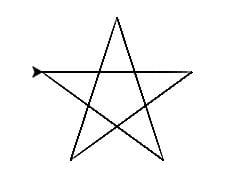# HSSlive: Plus One & Plus Two Notes & Solutions for Kerala State Board

## How to Draw a Star Using Python Turtle, Tkinter, Matplotlib & Without Libraries

How to Draw a Star in Python - There are various methods to create a Star using python libraries like turtle, tkinter and matplotlib. Also, you will learn how to make a Star using python in easy and simple steps. We have also provided the python code for drawing a Star pattern. If you have introductory to intermediate knowledge in Python and statistics, then you can use this article as a one-stop shop for building and plotting Star patterns in Python using libraries from its scientific stack, including NumPy, Matplotlib, Pandas, and Seaborn.

Plotting a Star in python is a simple procedure, and python in its simplest provides multiple easy methods to do so.

## How to Draw a Star using Python?

Given below is the process to draw a Star in python using libraries like Turtle, Tkinter, Matplotib and others.

Four Method to make a Star in Python using multiple libraries:

1. How to draw a Star using python turtle?
2. How to draw a Star using python tkinter?
3. How to draw a Star using python matplotlib?
4. How to draw a Star using python code without library?

## How to Draw a Star using Python Turtle?

Check below step by step process to make a Star using the python turtle library.

Steps

1. Define an instance for turtle.
2. For a drawing, a Star executes a loop 5 times.
3. In every iteration move the turtle 100 units forward and move it right 144 degrees.
4. This will make up an angle 36 degrees inside a star.
5. 5 iterations will make up a Star perfectly.

Code

 `#Program to draw stars in Python Turtle` `import` `turtle`   `t ``=` `turtle.Turtle()`   `for` `i ``in` `range``(``5``):` `    ``t.forward(``150``)` `    ``t.right(``144``)`

OutputHow to Draw a Star Using Python Turtle, Tkinter, Matplotlib & Without Libraries

## How to Draw a Star using Python Tkinter?

Check below step by step process to make a Star using the python tkinter library.

Step

Code

# coding:utf-8
#
from tkinter import *
import math

base=Tk()
canvas=Canvas(base,width=300,height=300,background='grey')
canvas.pack()

#
center_x=150
center_y=150
r=150 # The distance from the center point to each angle, called the radius

# Place the five points of the five-pointed star in turn
points=[
#
center_x-int(r*math.sin(2*math.pi/5)),
center_y-int(r*math.cos(2*math.pi/5)),

#
center_x+int(r*math.sin(2*math.pi/5)),
center_y-int(r*math.cos(2*math.pi/5)),

#
center_x-int(r*math.sin(math.pi/5)),
center_y+int(r*math.cos(math.pi/5)),

# vertex
center_x,
center_y-r,

#
center_x+int(r*math.sin(math.pi/5)),
center_y+int(r*math.cos(math.pi/5)),
]
# Create a polygon based on ten vertices
canvas.create_polygon(points,outline='green',fill='yellow')
# 's five-pointed star ‘three words at the center
Canvas.create_text(150,150,text='pentagram')
#
base.mainloop()

Output

## How to Draw a Star using Python Matplotlib?

Check below step by step process to make a Star using the python matplotlib library.

Step

Code

Output

## How to Draw a Star using Python Code without Library?

Step

Code

Output

Note: Also, these codes might not run in an online compiler please use an offline compiler. Programmers can make any changes in the code according to their specific requirement.

## Conclusion on How to Draw a Star using Python

We hope the programs and methods provided above on how to make a Star using python libraries have been helpful to you. If there is any issue in any of the code please let us know in the comments. We will try to resolve it as soon as possible.

Share: